By: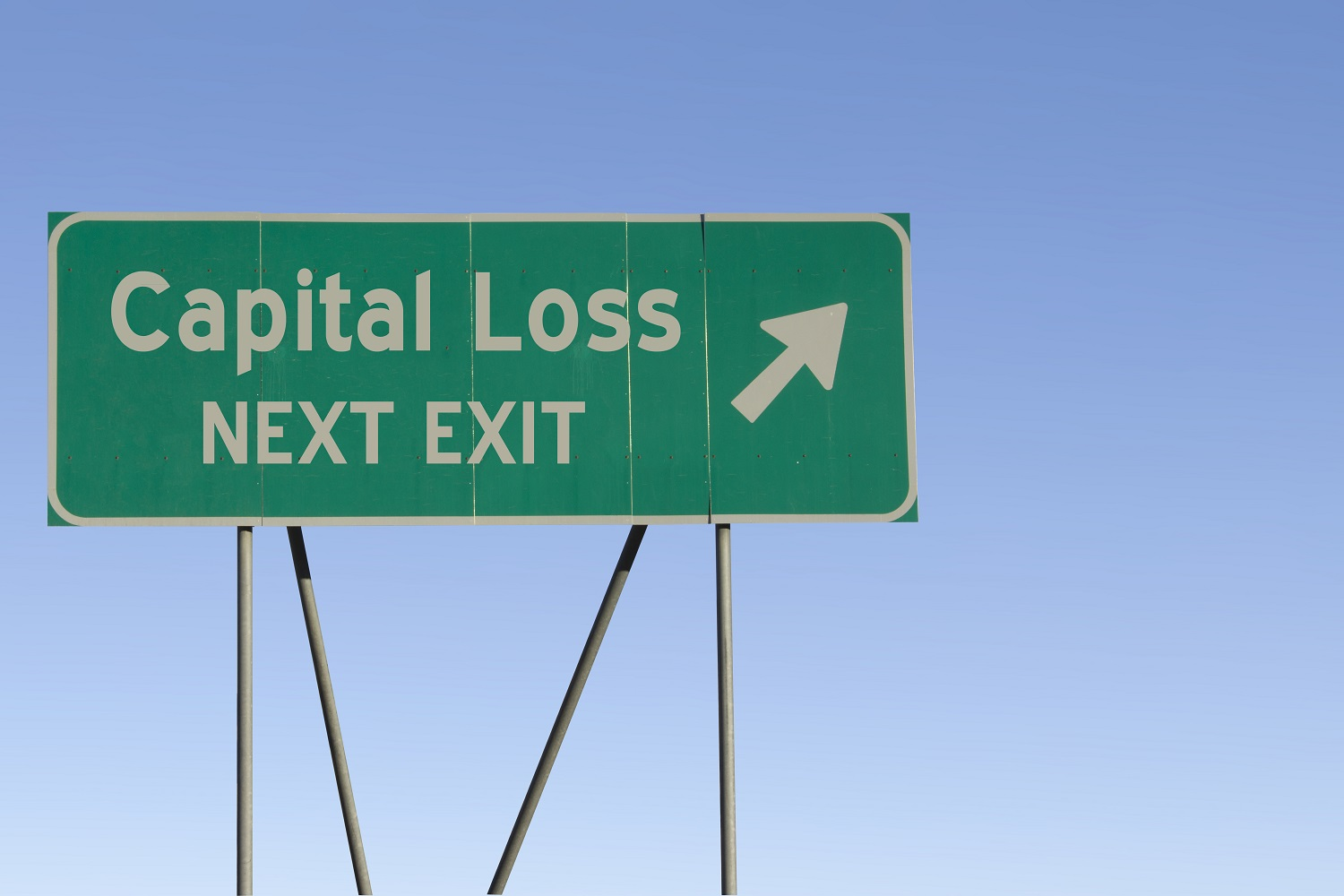Reprinted with permission from Schanel and Associates, CPA. www.schanelcpa.com. Original Post: October 04, 2019

When an investor sells a stock or mutual fund that produces a loss, this capital loss reduces taxable income by first offsetting any capital gains, and then by reducing taxable income up to \$3,000. Net capital losses in excess of \$3,000 generate what is called a capital loss carryforward that can be used in future tax years. Capital loss carryforwards can be very valuable, but the process of utilizing them is not always straightforward.

#### Loss Carryforwards Retain Their Character

To understand capital loss carryforwards, we start with the fact that long-term capital gains and short-term capital gains are taxed differently. When an investment is held for more than a year, the gain is taxed at a lower, capital gains tax rate, usually 15%. If the investment is held for less than a year, the gain is taxed as ordinary income tax rates, up to 37%.

Similarly, capital loss carryforwards must also be classified as either short-term or long-term. This is determined by the holding period for the investment that led to the loss carryforward.

#### Utilizing the Loss Carryforward

The use of a loss carryforward involves the following steps.

First, short-term loss carryforwards are netted against the current year’s short-term gains and losses, and long-term loss carryforwards are netted against the current year’s long-term gains and losses. If at that point there are no losses left, then the process stops there, and the net long-term gains are taxed at the capital gains rate and the net short-term gains are taxed at ordinary rates.

Second, if there are losses leftover in either or both categories, then both types of gains and losses are combined, and an overall gain or loss is calculated. During this process, there is no distinction between short-term and long-term loss carryforwards. Third, if the taxpayer is left with an overall loss of more than \$3,000, the amount of loss in excess of \$3,000 is re-segregated into either short-term and/or long-term losses and carried forward to the next year accordingly.

#### Short-Term Loss Carryforwards May Be More Valuable

In certain situations, short-term loss carryforwards are more valuable than long-term loss carryforwards.

For example, if an investor has realized short-term capital gains of \$10,000 and long-term capital gains of \$10,000, a short-term capital loss carryforward of \$5,000 will produce a long-term capital gain of \$10,000 and a short-term taxable gain of \$5,000. Assuming a marginal tax rate of 32% and a capital gains rate of 15% the investor will pay tax of \$1,600 + \$1,500 = \$3,100.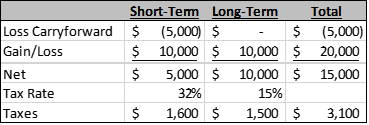However, if the investor instead had a long-term capital loss carryforward of \$5,000, the investor would be left with a \$10,000 short-term gain and a \$5,000 long-term gain.  The tax paid would be \$3,200 + \$750 = \$3,950.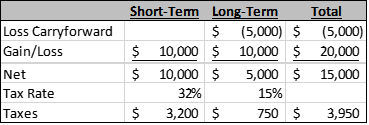The short-term loss carryforward produced a tax benefit of \$850 relative to a long-term loss carryforward.  Notice that in these two examples, the use of the loss carryforward stopped at step one because no losses were calculated in either category.

If, however, a loss is leftover, both types of gains and losses are combined into an overall gain or loss.  When this happens, a loss carryforward produces the same benefit regardless of whether its long-term or short-term.

For example, if an investor has a \$20,000 long-term capital gain and a \$5,000 loss carryforward, the \$5,000 is used to offset the long-term gain regardless of whether it’s a short-term loss carryforward or a long-term loss carryforward.

Below is the calculation if the loss carryforward is short-term: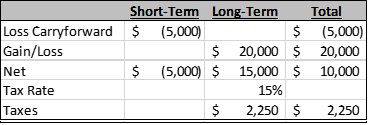And below is the calculation if the loss carryforward is long-term:

In both cases, the investor is left with a \$15,000 net long-term capital gain, which will be taxed at 15% for \$2,250 in taxes.

#####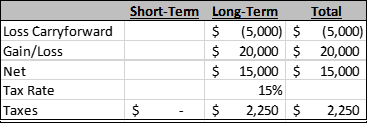As you can see, short-term loss carryforwards sometime produce more tax savings than long-term loss carryforwards, but not always.

So, what should you do as an investor?  All else being equal and where possible, choose short-term losses over long-term losses. They may produce a benefit in the future, and even if they don’t, you will not be worse off.